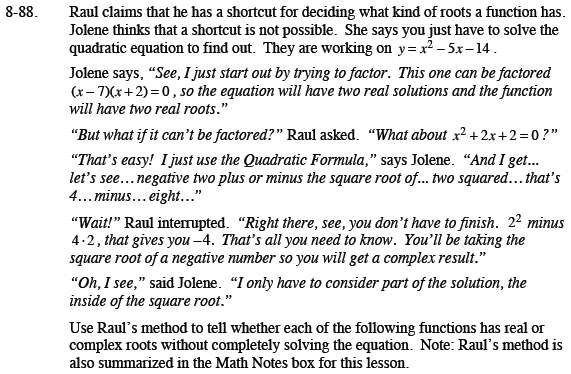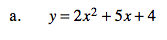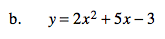Home > CCA2 > Chapter 8 > Lesson 8.2.2 > Problem8-88

8-88.
1. Raul claims that he has a shortcut for deciding what kind of roots a function has. Jolene thinks that a shortcut is not possible. She says you just have to solve the quadratic equation to find out. They are working on y = x2 − 5x − 14.

2. Use Raul’s method to tell whether each of the following functions has real or complex roots without completely solving the equation. Note: Raul’s method is also summarized in the Math Notes box for this lesson. Homework Help ✎

1. y = 2x2 + 5x + 4

2. y = 2x2 + 5x − 3$b^2-4ac$Calculate the discriminant to see if it is positive or negative.The discriminant is positive so the roots are real.